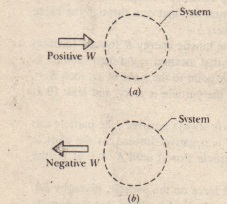# Work Done on a System by an External Force

Work Done on a System by an External Force

We defined work as being energy transferred to or from an object by means of a force acting on the object. We can now extend that definition to an external force acting on a system of objects.

Work is energy transferred to or from a system by means of an external force acting on that system.

These transfers are like transfers of money to and from a bank account. If a system consists of a single particle or particle-like object, the work done on the system by a force can change only the kinetic energy of the system. The energy statement for such transfers is’ the work – kinetic energy theorem of that is, a single particle has only one energy account. called kinetic energy. External forces can transfer energy into or out of that account. If a system is more complicated.

Let us find energy statements for such systems by examining two basic situations, one that does not involve friction and one that does.(a) Positive work Won an arbitrarysystem means a transfer of energyto the system. (b) Negative work.W means a transfer of energy from thesystem